# Time Trigger

A clock that only works when someone is in front of it. You are the only one who can trigger the clock to display the current time.

BeginnerFull instructions provided17 hours1,057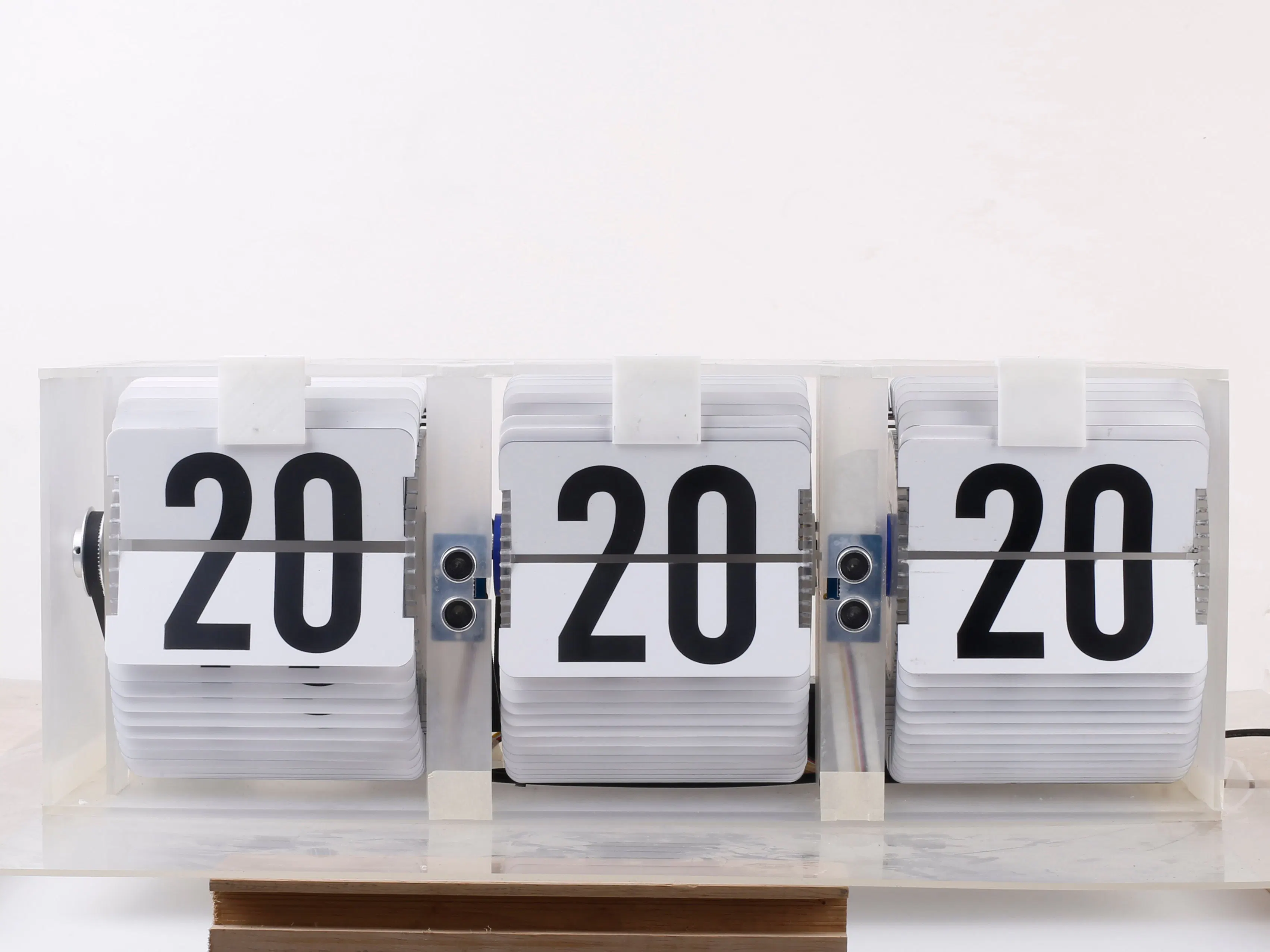## Things used in this project

### Hardware components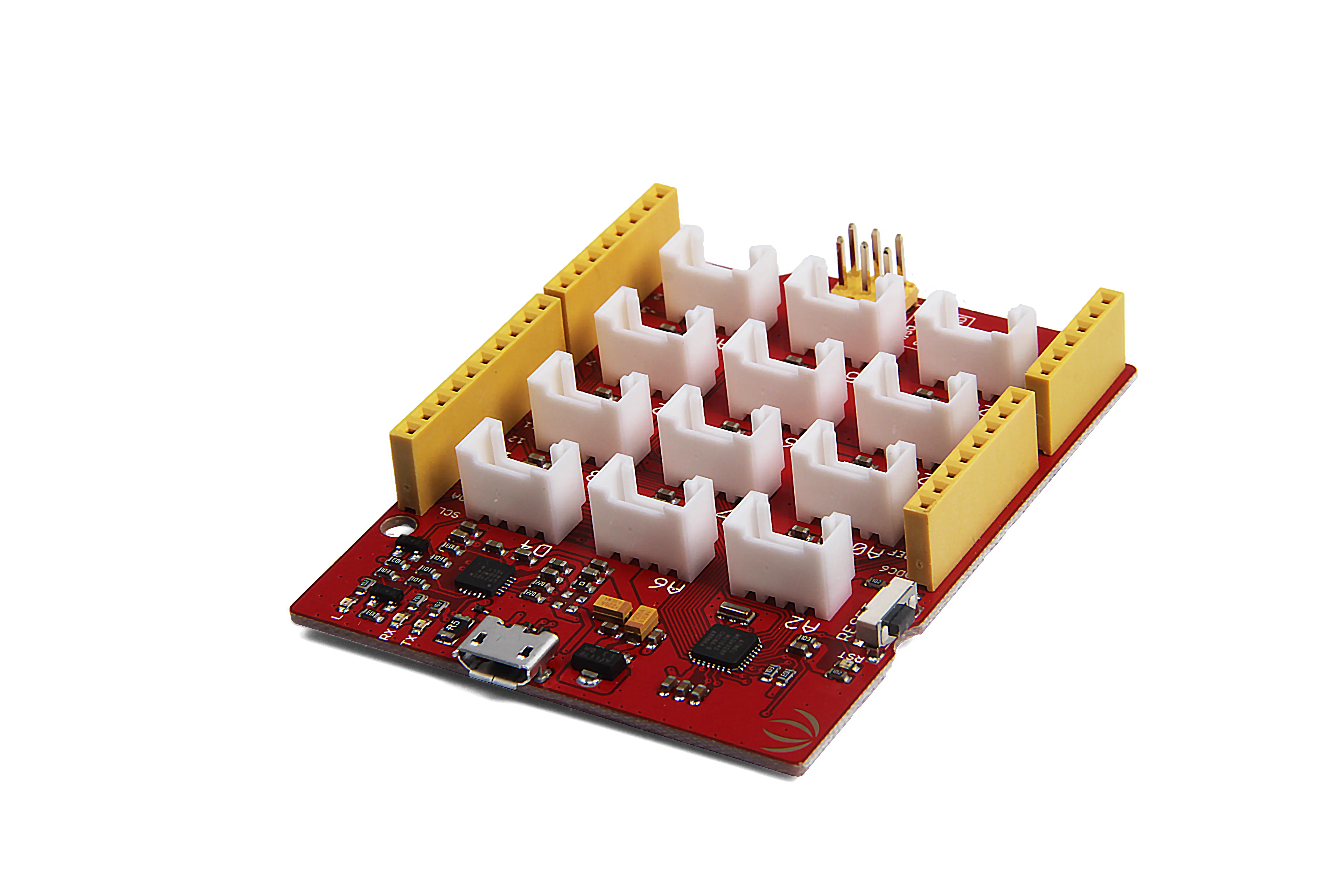Seeed Studio Seeeduino Lotus V1.1 - ATMega328 Board with Grove Interface
×1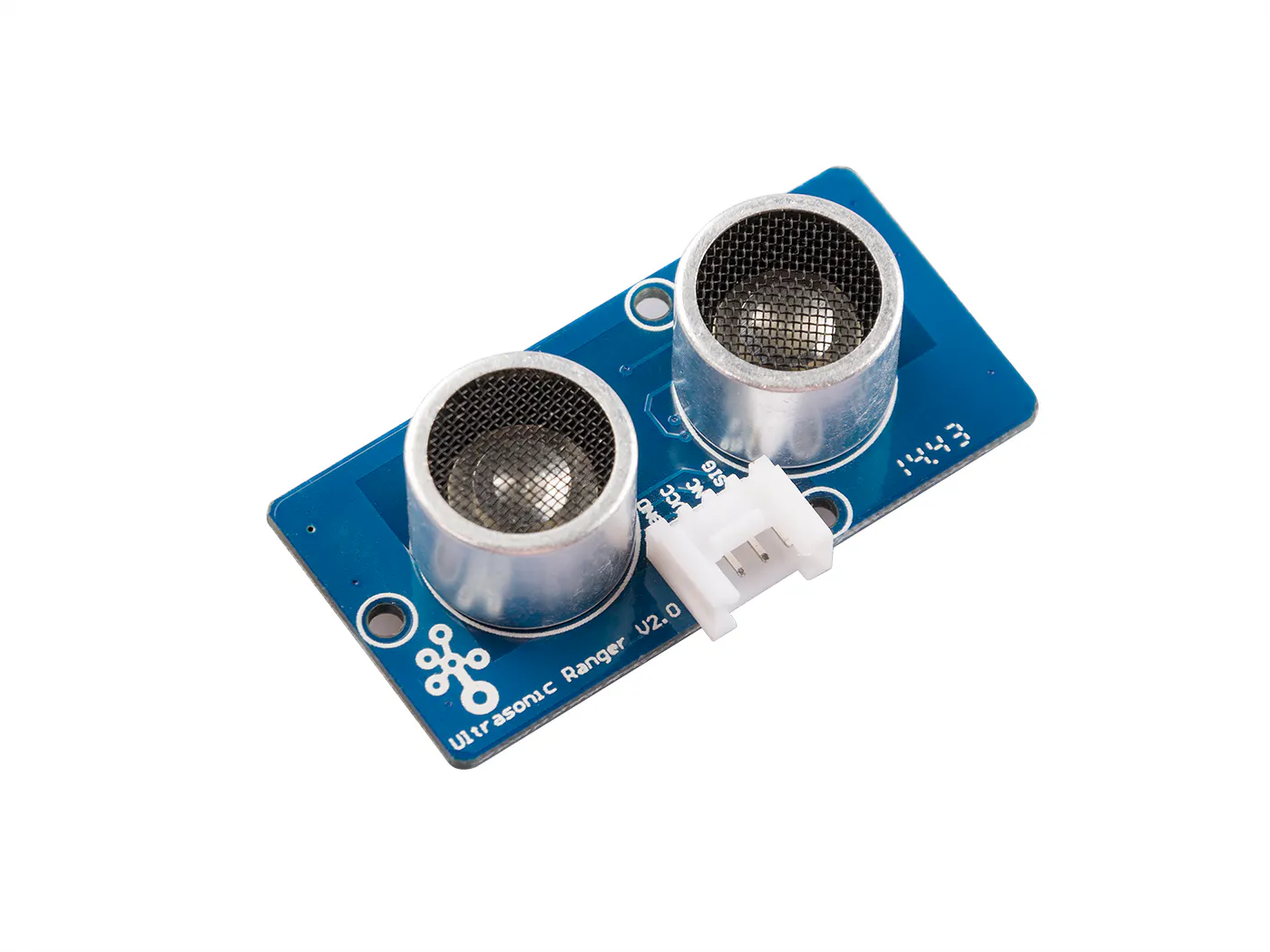Seeed Studio Grove - Ultrasonic Ranger
×1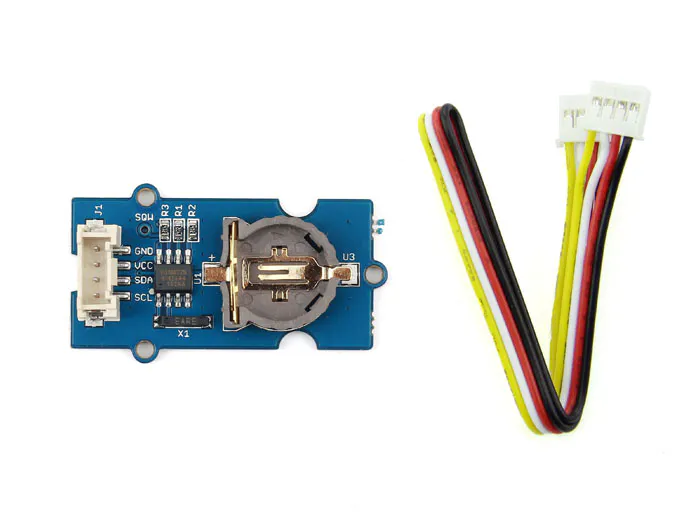Seeed Studio Grove - RTC
×1
 Step Motor 42BYGH47-401A
×1
 Stepper Motor Driver Board TB6560 TB6600
×3
 Timing Belt (6mm x 400mm)
×3
 Clear Transparent Frosted Acrylic (1m x 1m x 0.04m)
×1
 White Acrylic (1m x 1m x 0. 2m)
×1
 20-teeth Synchronous Wheel (internal diameter 5mm, length 6mm)
×3
 60-teeth Synchronous Wheel (internal diameter 5mm, length 6mm)
×3
 Screws, generic
×6
 Nuts, generic
×3
 3D-printed parts
×6
 Black Matte Spray Paint
×1
×1
×1
 Glue
×1Jumper wires (generic)
×1

### Software apps and online servicesArduino IDE

### Hand tools and fabrication machines3D Printer (generic)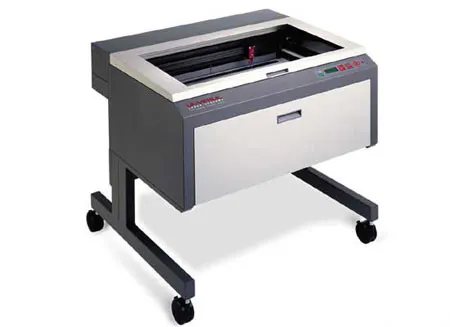Laser cutter (generic)

## Code

### TIme Trigger

Arduino
```#include <DRV8825.h>
#include "Ultrasonic.h"
#include <FlexiTimer2.h>
#include <DS1307.h>

#define STEP1 8
#define STEP2 9
#define STEP3 10

#define DIR1 5
#define DIR2 6
#define DIR3 7

Ultrasonic ultrasonic1(2);
Ultrasonic ultrasonic2(3);

DS1307 clock;//define a object of DS1307 class

int pre_h = 0;
int pre_m = 0;
int pre_s = 0;

int delta_h = 0;
int delta_m = 0;
int delta_s = 0;

bool s_run = 0;
bool m_run = 0;
bool h_run = 0;
bool run_flag = 0;

void step(int n,int pin)
{
for(int i=0;i<n;++i)
{
digitalWrite(pin,HIGH);
delayMicroseconds(200);
digitalWrite(pin,LOW);
delayMicroseconds(200);
}
}

void test()
{
step(320,STEP1);
step(320,STEP2);
step(320,STEP3);
}

void Clock()
{
if(run_flag == 1)
{
delta_s = clock.second - pre_s;
delta_m = clock.minute - pre_m;
delta_h = clock.hour - pre_h;
if(delta_s<0) delta_s += 60;
if(delta_m<0) delta_m += 60;
if(delta_h<0) delta_h += 60;

step(320*delta_s,STEP1);
step(320*delta_m,STEP2);
step(400*delta_h,STEP3);

pre_h += delta_h;
pre_m += delta_m;
pre_s += delta_s;

if(pre_h>=60) pre_h %= 60;
if(pre_m>=60) pre_m %= 60;
if(pre_s>=60) pre_s %= 60;

delta_s = 0;
delta_m = 0;
delta_h = 0;

run_flag = 0;
}
}

void setup()
{
Serial.begin(115200);
pinMode(STEP1,OUTPUT);
pinMode(STEP2,OUTPUT);
pinMode(STEP3,OUTPUT);
pinMode(DIR1,OUTPUT);
pinMode(DIR2,OUTPUT);
pinMode(DIR3,OUTPUT);
digitalWrite(DIR1,HIGH);
digitalWrite(DIR2,HIGH);
digitalWrite(DIR3,LOW);
pre_h = 0;
pre_m = 0;
pre_s = 0;
run_flag = 1;
clock.begin();
clock.getTime();
FlexiTimer2::set(10, 1.0/1000, Clock);
FlexiTimer2::start();
}

void loop()
{
clock.getTime();
ultrasonic1.MeasureInCentimeters();
ultrasonic2.MeasureInCentimeters();
if(ultrasonic1.RangeInCentimeters<=60||ultrasonic2.RangeInCentimeters<=60)
{
run_flag = 1;
}else
{
run_flag = 0;
}
}
```